# How to use Cross-WLF viscosity model in Polyflow

Hi,

How can I import the Cross-WLF viscosity model into Polyflow. In Polyflow, I can only select Cross model for shear rate dependence and WLF model for temperature dependence individually. The equation for Cross-WLF is different from Cross and WLF individually.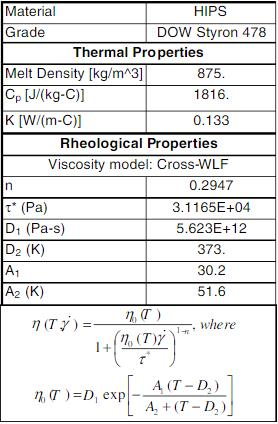Cross-WLF formula,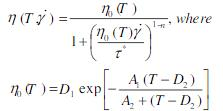Cross formula in Polyflow,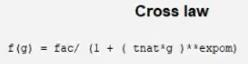WLF formula in Polyflow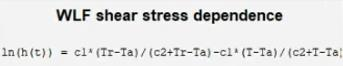My current approach to combine the equations was to assign the following parameters,

for Cross law,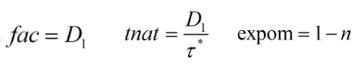and select the WLF shear stress dependence because,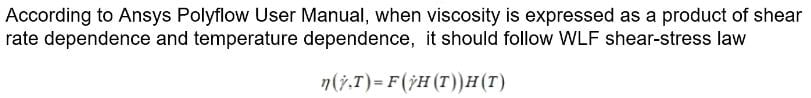finally for WLF shear stress dependence parameters, to match it to requirements I assign, Tr - Ta = 0 to get,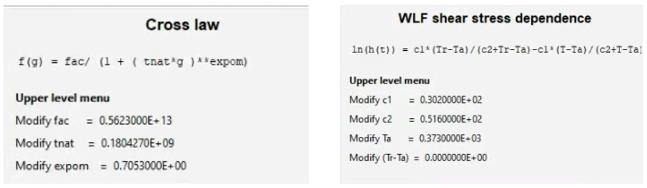Is this the correct approach?